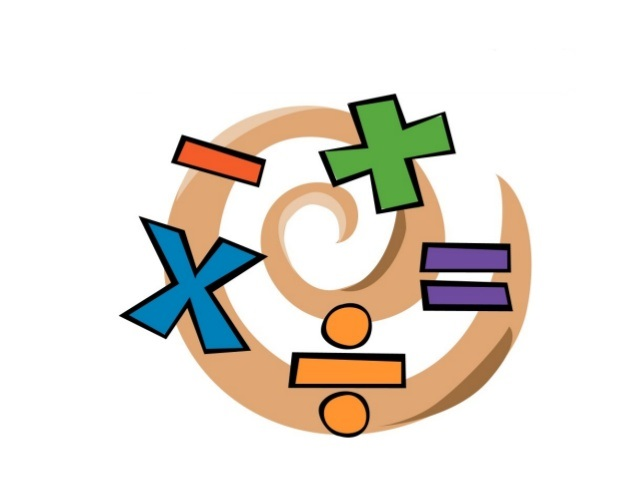# JavaScript #6 ~ Basic Operators

hjbaek91·2021년 5월 21일
0

## [Learn] JavaScript

목록 보기
7/20Today is a bit of recap on basic operators used in JavaScript with some examples. Once we have our values in our variables, what can we do with them? We can manipulate JavaScript values to combine or transoform into completely new values through basic operators. There are many different types of operators that are used in JavaScript in different situations. Some of these include: mathematical, comparison, logical, assignment, and so on.

Mathematical Operator

// Numbers
const now = 2021
const ageJohn = now - 1998;
const ageSarah = now - 2001;
console.log(ageJohn); // 23
console.log(ageSarah); // 20
console.log(ageJohn * 2); // 46
console.log(ageJohn / 10); // 2.3
// Strings
const firstName = 'John';
const lastName = 'Smith';
console.log(firstName + ' ' + lastName); // John Smith

Assignment Operator

// equal sign used in for variables and values
let x = 10 + 5; // 15
x += 10; // x = x + 10 = 25
x *= 4; // x = x * 4 = 100
x++; // x = x + 1;
x--; // x = x - 1;
console.log(x); // 99

Comparison Operator

// boolean (>, <, >=, <=)
const now = 2021
const ageJohn = now - 1998;
const ageSarah = now - 2001;
console.log(ageJohn > ageSarah); // true
console.log(ageSarah >= 18); // true
const now = 2021
const ageJohn = now - 1998;
const ageSarah = now - 2001;
console.log(now - 1998 > now - 2001);